# celestial sphere

Also found in: Dictionary, Thesaurus, Medical, Wikipedia.
Related to celestial sphere: Celestial coordinate system

## celestial sphere

celestial sphere, imaginary sphere of infinite radius with the earth at its center. It is used for describing the positions and motions of stars and other objects. For these purposes, any astronomical object can be thought of as being located at the point where the line of sight from the earth through the object intersects the surface of the celestial sphere. In astronomical coordinate systems, the coordinate axes are great circles on the celestial sphere. In most systems of this type, the reference points are fixed on the sphere, so the two coordinates needed to locate a body are relatively constant.

## celestial sphere

The sky considered as the inside of an imaginary sphere of indeterminate but immense radius that surrounds the Earth. It provides a convenient surface on which to draw and study the directions and motions of celestial bodies and other points in the sky. The Earth's west-to-east rotation causes an apparent rotation of the celestial sphere about the same (extended) axis once every 24 hours but in an east-to-west direction.

The measurements that may be made with the celestial sphere, using the four main coordinate systems of positional astronomy, concern not the distances but only the directions of celestial bodies. Angular relationships are established by the methods of spherical trigonometry (see spherical triangle). The principal reference circles on the celestial sphere are the celestial equator, the ecliptic, and an observer's horizon, which are all great circles. The principal points are the poles of these circles and the points of intersection – the equinoxes and cardinal points – of the circles (see illustration).

Collins Dictionary of Astronomy © Market House Books Ltd, 2006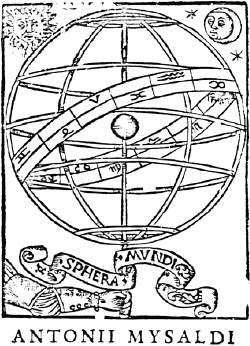A diagram of the celestial sphere from Orontius Fine, c. 1542. Reproduced by permission of Fortean Picture Library.

## Celestial Sphere

(religion, spiritualism, and occult)

The celestial sphere can be understood by imagining that all the objects in the sky are stuck against the inside of a gigantic hollow sphere, with Earth located at the exact center. The basic notion of the celestial sphere is assumed in various systems for locating celestial bodies in terms of celestial coordinates. Very much like terrestrial coordinates, which involve specifying a location in terms of longitude and latitude, celestial coordinates require two measurements of distance, expressed in terms of degrees of a circle. The altitude-azimuth system begins by situating itself at a specific location on the Earth’s surface, and uses the horizon, the zenith, and the north-south axis as points of reference. The equatorial system uses the celestial equator (Earth’s equator extended out into space and projected against the backdrop of the celestial sphere) and the vernal point (where the Sun is located at the point of the spring equinox) as its points of reference. Astronomers most often use the equatorial system. The ecliptic system uses the ecliptic (the orbit of Earth around the Sun, projected outward against the celestial sphere) and the vernal point. Astrologers use the ecliptic system.

### Sources:

Brau, Jean-Louis, Helen Weaver, and Allan Edmands. Larousse Encyclopedia of Astrology. New York: New American Library, 1980.
Filbey, John, and Peter Filbey. The Astrologer’s Companion. Wellingborough, Northamptonshire, UK: Aquarian Press, 1986.
The following article is from The Great Soviet Encyclopedia (1979). It might be outdated or ideologically biased.

## Celestial Sphere

an imaginary sphere of arbitrary radius on which celestial objects are projected; it is used for the solution of various astrometric problems. The concept of the celestial sphere originated in remote antiquity; it was based on the visual impression of a dome-shaped celestial surface. This impression results from the enormity of the distances to celestial objects; the human eye is unable to estimate differences in such distances, so that all the objects seem uniformly distant. Ancient peoples associated this with the presence of a real sphere that bounds the whole world and has numerous stars set in its surface. Thus, according to their concept, the celestial sphere was a most important element of the universe. With the growth of scientific knowledge, this concept of the celestial sphere lost importance. However, the geometry established in antiquity for the celestial sphere had already been developed and perfected into a modern form, in which it continues to be used in astrometry.

The radius of the celestial sphere may be taken to be whatever is convenient; in order to simplify geometrical relations, it is taken equal to unity. The type of problem dictates where the center of the celestial sphere is to be located; at the position of the observer (topocentric celestial sphere), at the center of the earth (geocentric celestial sphere), at the center of a planet (planetocentric celestial sphere), at the center of the sun (heliocentric celestial sphere), or at any other point in space. To each celestial object there corresponds a point on the celestial sphere that is the point at which the straight line connecting the center of the celestial sphere and the center of the object intersects the celestial sphere. In the study of the relative positions and apparent motions of objects on the celestial sphere, some particular coordinate system is chosen. (See.) The coordinate system is defined by primary points and lines. The latter are usually great circles of the celestial sphere. Each great circle has two poles that are defined as the endpoints of the diameter perpendicular to the plane of the given circle.

Figure 1 depicts the celestial sphere as seen by an observer situated at a point on the earth’s surface with latitude Ф. A plumb line (vertical) drawn through the center of this sphere intersects the celestial sphere at points Z and Z’, called the zenith and nadir, respectively. The plane that passes through the center of the celestial sphere perpendicular to the vertical intersects the sphere along the great circle NESW, called the mathematical (or true) horizon. The mathematical horizon divides the celestial sphere into a visible hemisphere and an invisible hemisphere; the zenith is located in the former, and the nadir in the latter. The line that passes through the center of the celestial sphere parallel to the earth’s axis of rotation is called the polar axis, and it intersects the celestial sphere at the north and south celestial poles P and F, respectively. The plane that passes through the center of the celestial sphere perpendicular to the polar axis intersects the sphere along the great circle AWA’E, called the celestial equator. From the construction it follows that both the angle between the polar axis and the plane of the mathematical horizon and the angle between the vertical and the plane of the celestial equator are equal to the latitude Ф of the point of observation. The great circle that passes through the celestial poles, the zenith, and the nadir is called the celestial meridian.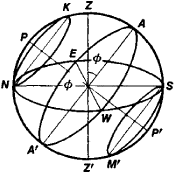Figure 1. Celestial sphere: (Z) zenith; (Z1) nadir; (NESW) mathematical horizon; (N), (£), (S), and (W) north, east, south, and west points; (P) and (P1) north and south celestial poles; (AWA1E) celestial equator; (Ф) geographic latitude

The celestial meridian intersects the horizon at two points—the one nearest the north celestial pole being called the north point N, and the one diametrically opposite being called the south point S. The line NS that passes through these points is the meridian line. The points on the horizon situated at 90” from the points N and S are the east point E and the west point W. The points N, E, S, and W are called the principal points of the horizon. The planes of the mathematical horizon and of the celestial equator intersect along the diameter EW.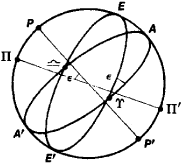Figure 2.

Note to Figure 2. Celestial sphere: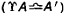celestial equator,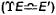ecliptic, (r) and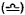vernal and autumnal equinoxes, (E) and (E’) summer and winter solstices, (P) and (P’) north and south celestial poles, (IT) and (Π’) north and south ecliptic poles

The great circle on the celestial sphere that the sun traces out during its apparent annual motion is called the ecliptic (Figure 2). The plane of the ecliptic forms an angle € = 23°27’ with the plane of the celestial equator. The ecliptic intersects the equator at two points, one of which is the vernal equinox (at which the sun in its apparent annual motion crosses from the southern celestial hemisphere into the northern celestial hemisphere), and the other, the autumnal equinox, which is diametrically opposite to the vernal equinox. The points on the ecliptic situated at 90° from the vernal and autumnal equinoxes are called the summer solstice and the winter solstice; the former is in the northern celestial hemisphere and the latter is in the southern celestial hemisphere. The great circle that passes through the celestial poles and the equinoctial points is called the equinoctial colure, and the great circle that passes through the celestial poles and the solstitial points is called the solstitial colure. When drawn on a star map, these circles cut off the tails of the ancient representations of the constellations Ursa Major (equinoctial colure) and Ursa Minor (solstitial colure).

The apparent diurnal displacement of the stars, which is a reflection of the real rotation of the earth about its axis, corresponds to a rotation of the celestial sphere about its polar axis with a period equal to one sidereal day. Because of the rotation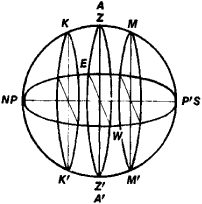Figure 3. Representation of celestial sphere at equator (ø = 0°)

of the celestial sphere, all representations of objects on it describe circles in space that are parallel to the equator, called diurnal circles. Depending on the position of the diurnal circles with respect to the horizon, three types of celestial objects are distinguished: (1) objects that do not set (diurnal circles located entirely above the horizon), (2) objects that do not rise (diurnal circles entirely below the horizon), and (3) objects that rise and set (diurnal circles intersecting the horizon). The diurnal circles KN and SM, which are tangent to the horizon at points N and S, respectively (Figure 1), are the boundaries for these groups of celestial objects. Since the visibility of a celestial object is determined by the position of the horizon, whose plane is perpendicular to the vertical, the conditions for visibility of celestial objects are different for points on the earth’s surface having different latitudes Ф. This phenomenon, already known in antiquity, has served as one proof of the spherical shape of the earth. On the equator (Ф =0°), the polar axis PP is located in the plane of the horizon and coincides with the meridian line NS. The diurnal circles (KK, MM) of all celestial objects intersect the plane of the horizon at right angles. In this case, all celestial objects rise and set (Figure 3). The angle between the polar axis and the horizon increases as the observer moves along the earth’s surface from the equator to the pole. More and more objects cease to set or to rise. At the pole (Ф = 90°), the polar axis coincides with the vertical, and the equatorial plane coincides with the plane of the horizon. In this case, each celestial object either never sets or never rises, since the diurnal circles (KK, MM) are situated in planes parallel to the horizon (Figure 4).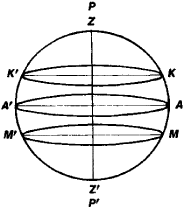Figure 4. Representation of celestial sphere at pole (ø = 0°)

### REFERENCES

Blazhko, S. N.Kurs sfericheskoi astronomii. Moscow-Leningrad, 1948.
Kazakov, S. A.Kurs sfericheskoi astronomii, 2nd ed. Moscow-Leningrad, 1940.

V. P. SHCHEGLOV

## celestial sphere

[sə′les·chəl ′sfir]
(astronomy)
An imaginary sphere of indefinitely large radius, which is described about an assumed center, and upon which positions of celestial bodies are projected along radii passing through the bodies.
McGraw-Hill Dictionary of Scientific & Technical Terms, 6E, Copyright © 2003 by The McGraw-Hill Companies, Inc.

## celestial sphere

An imaginary sphere of infinite radius, centered at the center of the earth, on which the apparent motion of celestial bodies takes place. Because all stars are so far from an earthbound observer that they can be located solely by their direction, it is convenient to presume they are located on the inside of a spherical surface centered on the observer. Because the radius of earth is negligible, it is convenient to consider earth, rather than the terrestrial observer, centered in the celestial sphere. Stars are designated by their location on this sphere, and planets and other heavenly bodies are located against this background.

## celestial sphere

an imaginary sphere of infinitely large radius enclosing the universe so that all celestial bodies appear to be projected onto its surface
Collins Discovery Encyclopedia, 1st edition © HarperCollins Publishers 2005
References in periodicals archive ?
The celestial sphere is moved by [its] soul (nafs), the soul being its proximate principle of motion.
But, since the celestial sphere is moving westward at 15[degrees] per hour, the big trick in celestial navigation is knowing your time.
equinox Either of the two points on the celestial sphere where the celestial equator intersects the ecliptic (i.e.
As they walk through, visitors will encounter the earliest known celestial sphere, found in eastern Turkey and dating back to 2nd century BC, as well as the scientific treatises by Greco-Roman astronomer, Claudius Ptolemy.
Celestial sphere. Unlike, say, "visualize invisible," the two terms thrust together in these examples are not inherently mutually exclusive.
The motion of the stars could be explained by the celestial sphere moving around us.
On Saturday, South Africa's powerful MeerKAT radio telescope, which is still under construction, gave a taste of things to come when it released its "first-light" image showing, in unprecedented detail, a patch of sky covering less than 0.01 percent of the entire celestial sphere. The image revealed 1,300 galaxies in this tiny corner of the universe where just 70 had been seen before.
Morsels V contains 69 chapters divided into six categories: The Moon, Eclipses and Occultations, Planetary Motions, Planetary Phenomena, On the Celestial Sphere and Varia.
What is the brightest star in our celestial sphere? 4.
Uttarayana is the time when the Sun travels towards north on the celestial sphere. The starting of Uttarayan is celebrated as Makar Sankranti throughout India.
This unusual and detailed encyclopedia reviews the widely known constellations and locates stars relative to Earth and the celestial sphere. Kaler, professor emeritus of astronomy at the University of Illinois, details the colors, spectra, and chemical composition of stars, the motion of the galaxy, and the forms of star groupings, including binary stars and clusters.

Site: Follow: Share:
Open / Close